# Identify the odd one out using counting methods

In this worksheet, students will identify an image that does not fit in with the rest of the group. The focus will be on counting, which could involve counting sides, shapes or lines.Key stage:  KS 2

Curriculum topic:  Spatial and Non-Verbal Reasoning

Curriculum subtopic:  Odd One Out

Difficulty level:### QUESTION 1 of 10

Welcome shape sorter.

We’re in a bit of a tricky situation, our shape sorting robot seems to have malfunctioned and not sorted the shapes correctly.

Could you help us find the odd one out and help solve this puzzle?

Which of the shapes below is the odd one out and doesn’t fit well with the others?

a)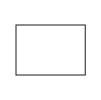b)c)d)e)First, we need to look for something that four of the shapes have in common.

It is always a good idea to count the number of sides first.

Shapes a, b, c and e all have four sides and we call these shapes quadrilaterals.

So shape d is the odd one out as it has five sides.

Let’s try another one together:

Which shape below is the odd one out?

a)b)c)d)e)First, we need to look for something that four of the shapes have in common.

All of the shapes are circles with squares inside.  It’s always a good idea to see if there is anything we can count in this type of question. What could we count?

If you count the number of squares, you will notice that shapes a, b, c and d all have five squares inside them.

This means that shape e is the odd one out as it has only four squares in the middle not five.

It’s now your turn to identify the odd shape.

The theme is counting so always be on the lookout for what you can count.

Good luck shape sorter!

Which of the shapes below is the odd one out and doesn't fit well with the others?

a)b)c)d)e)Which of the shapes below is the odd one out and doesn't fit well with the others?

a)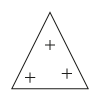b)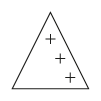c)d)e)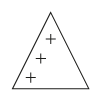Which of the shapes below is the odd one out and doesn't fit well with the others?

a)b)c)d)e)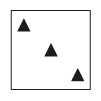Which of the shapes below is the odd one out and doesn't fit well with the others?

a)b)c)d)e)Which of the shapes below is the odd one out and doesn't fit well with the others?

a)b)c)d)e)Which of the shapes below is the odd one out and doesn't fit well with the others?

a)b)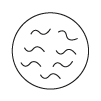c)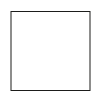d)e)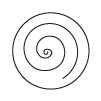Which of the shapes below is the odd one out and doesn't fit well with the others?

a)b)c)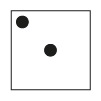d)e)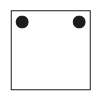Which of the shapes below is the odd one out and doesn't fit well with the others?

a)b)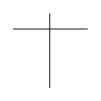c)d)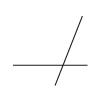e)Which of the shapes below is the odd one out and doesn't fit well with the others?

a)b)c)d)e)Which of the shapes below is the odd one out and doesn't fit well with the others?

a)b)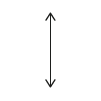c)d)e)• Question 1

Which of the shapes below is the odd one out and doesn't fit well with the others?

c)EDDIE SAYS
Counting is really important in this question. If we count the number of sides that each shape has, we can see that options a, b, d and e all have four sides. Option c has six sides - how greedy! This means that option c must be the odd one out. Remember that all four-sided shapes are called quadrilaterals.
• Question 2

Which of the shapes below is the odd one out and doesn't fit well with the others?

d)EDDIE SAYS
This is another question where counting is important. All of the outside shapes are the same, so the difference must be in what is inside them. Options a, b, c and e all have three + signs inside them, whilst option d only has two. This means that option d must be the odd one out. I'm counting on you to COUNT carefully in these questions!
• Question 3

Which of the shapes below is the odd one out and doesn't fit well with the others?

e)EDDIE SAYS
Engage the counting part of your brain detective! Here we can see five squares with shapes inside. Most of the squares have two black triangles inside them. Sneaky square e has one triangle too many! This means that option e must be the odd one out.
• Question 4

Which of the shapes below is the odd one out and doesn't fit well with the others?

b)EDDIE SAYS
At first glance, all of these shapes look totally different! Let's look for something to count here... If we count the number of sides, we can see that options a, c, d and e all have five sides and option b only has four. This means that option b must be the odd one out.
• Question 5

Which of the shapes below is the odd one out and doesn't fit well with the others?

c)EDDIE SAYS
I'm afraid that counting doesn't really help in this question! If we look inside the shapes, we can see that four of them have lines going across (horizontal) and down (vertical). Only option c has no lines going across. It still has lines going down, but this feature makes it the odd one out.
• Question 6

Which of the shapes below is the odd one out and doesn't fit well with the others?

c)EDDIE SAYS
Option c is the odd one out here as it is a square and all the others are circles. The pattern inside the circles was trying to catch us out and make us second guess our answer - don't be distracted, as this pattern doesn't matter at all. Watch out for red herrings like these detective!
• Question 7

Which of the shapes below is the odd one out and doesn't fit well with the others?

d)EDDIE SAYS
The question wanted us to count again. We needed to count how many circles were inside each square. Squares a, b, c and e all have two circles but option d only has one, so it is the odd one out. Were you able to SPOT that one?
• Question 8

Which of the shapes below is the odd one out and doesn't fit well with the others?

e)EDDIE SAYS
Don't get cross-eyed with these crossed lines! Options a, b, c and d all have two lines which are crossing, whilst poor option e only has one line, so this must be our odd one out.
• Question 9

Which of the shapes below is the odd one out and doesn't fit well with the others?

e)EDDIE SAYS
We have some more tricky patterns trying to distract us here! Don't be distracted, as the patterns don't matter. Options a, b, c and d are all triangles, whilst option e is a circle. So option e does not fit well with the others and is the odd one out. Well done for ignoring those distracting lines and dots which have been put in to fool us!
• Question 10

Which of the shapes below is the odd one out and doesn't fit well with the others?

a)